# Cubes and Cuboids

Cubes and cuboids are an integral part of many public sector exams. It’s a part of the logical reasoning section of aptitude. The study of these topics helps in improving analytical and logical skills. The topics require pictorial skills to solve the problems. The right diagram comes from the correct imagination and analysis.

Following is the list of exams where one can find the questions based on cubes and cuboids.

Exams List:

To explore various SBI Exams at length, check at the linked article.

Topics list:

1. Basic 3-D structure of a cube.
2. Surface area and volume of a cube and cuboid
3. Creation of Rubik’s cube
4. Evaluation of Cube with ‘n’ sides painted
5. Miscellaneous problems## Basic Structure of a Cube

A cube is a 3-dimensional structure with three sides (length, width, and height) where all the sides equal (length = width= height). The word cube is derived from the Arabic word ”Kaba” a large cube-shaped structure.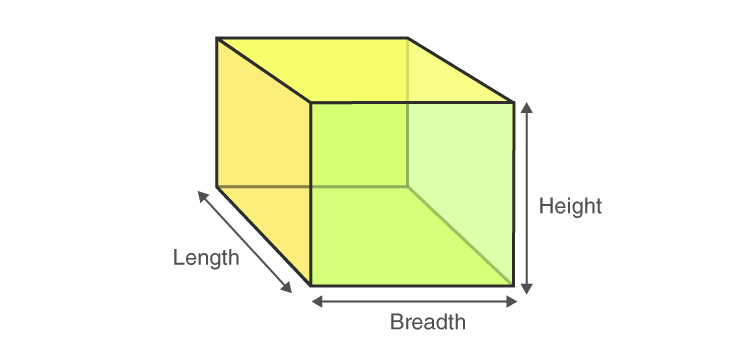A cube has 6 faces: The faces appear at front, back, right, left, top and bottom.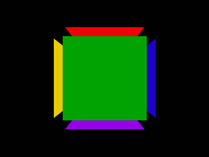Cube has 8 vertices: The corner points are called vertices. There are four vertices on the top face and four more at the bottom face.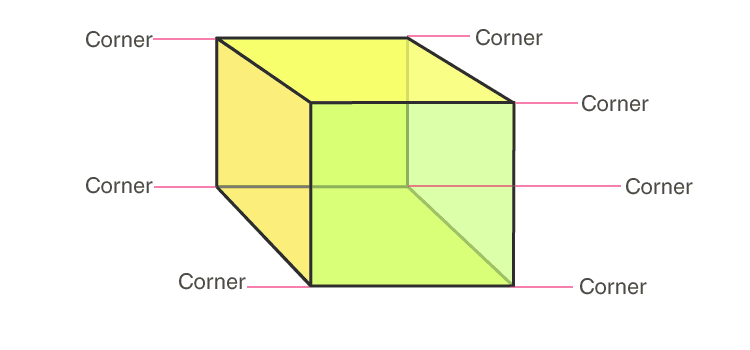Cube has 12 Edges:

The line which connects the two vertices is called edges. There are twelve edges in a cube. Four on the top surface and four at the bottom and four more verticle lines connecting the opposite vertices of top and bottom face.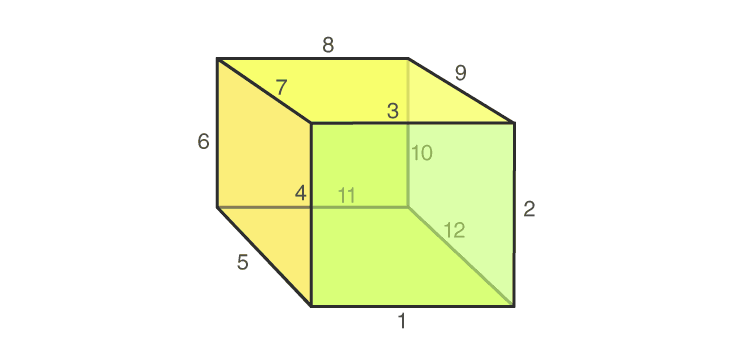Summary:

 A cube has: Faces Vertices Edges 6 8 12

To explore Logical Reasoning in detail, check at the linked article.

### Surface Area of Cube

A cube is a 3-dimensional representation of a square. Since dimensions of all the three sides, i.e. length, breadth, and height of a cube are equal, they are referred to as sides and is indicated by a symbol ‘s’. The total surface area of a cube is:
The surface area of a cube = (area of one square) *6

The surface area of a cube = (s*s)*6

The surface area of a cube = 6

The sum of areas of 4 constituting squares (faces) gives the lateral surface area of the cube.

The lateral surface area of a cube = 4*(area of one square)

The lateral surface area of a cube = 4*(s*s)

The lateral surface area of a cube = 4

### What is Cuboid?

A cuboid is a 3-dimensional structure with three sides where all the sides are not equal. The three sides are the length, width, and height. All of its faces are rectangles A cuboid also has 6 faces, 8 vertices, and 12 edges.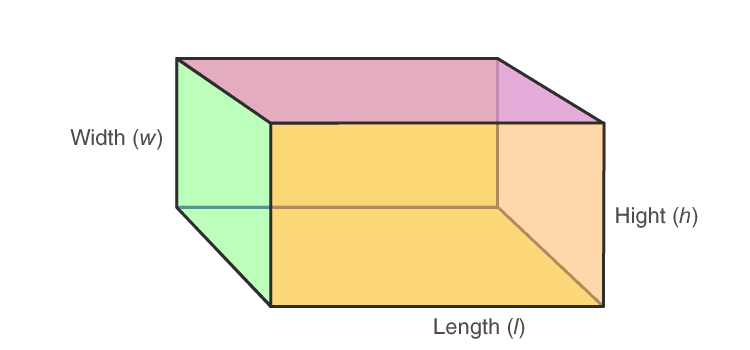The total surface area (TSA) of a cuboid is the sum of the areas of its 6 faces:

To explore Directions in detail, check at the linked article.

Lateral Surface Area of Cuboid

The lateral surface area of a cuboid is the sum of the area of only four rectangles.

Lateral Surface Area of Cuboid = Area of left side face +

Area of right side face +

Area of front Face +

Area of back Face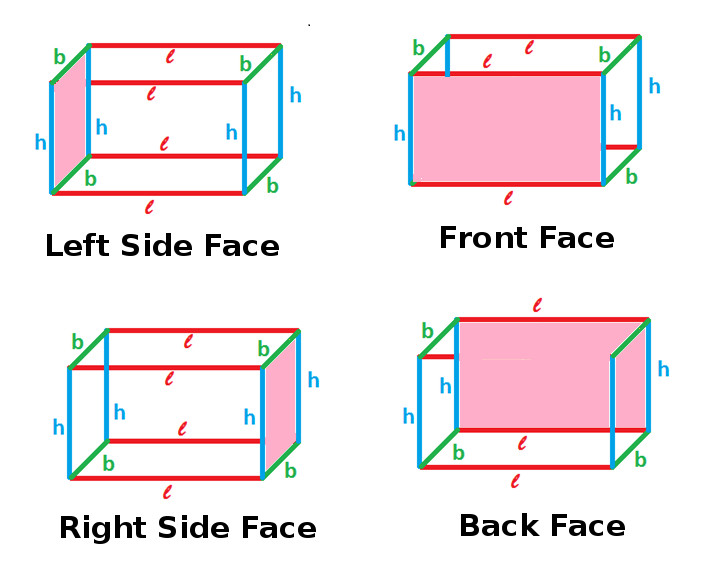The lateral surface area of a cuboid is given by:

Formulae table:

 Cube Cuboid TSA = 6 LSA = 4

To explore Calendars in detail, check at the linked article.

### Creation of Rubik’s cube

If we divide a cube into the size part of its side, we get smaller cubes. A cube Shown below, which is painted on all the sides and then cut into 1/4th of its original face. Each small cube is known as “unit cube”.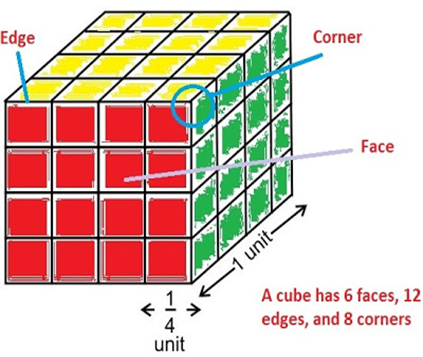The total number of smaller(unit) cubes generated due to the division of a cube to its .

Ex: If we cut a cube to form three rows and three columns on each face then the total number of unit cubes will be = 3*3*3 = 27.

Following is the list of unit cube generation based on the division of a bigger cube:

 Rows on each side Columns on each side Unit cubes 2 2 8 3 3 27 4 4 64 5 5 125 6 6 216 7 7 343

To explore Seating Arrangement in detail, check at the linked article.

### Evaluation of Cube with ‘n’ sides painted

Observe the cube shown. It has been divided into ¼ of its original side length. Hence the total number of smaller or unit cubes formed will be 64.

Question 1: How many unit cubes have only three sides painted?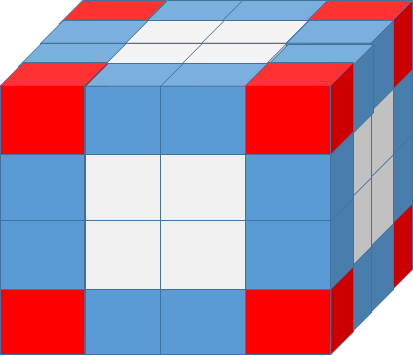Solution:

The cubes with three of their sides painted lie at the vertices of the cubes (The cubes coloured in red). There are eight such cubes. Hence the answer is eight.

Question 2: How many cubes have only two sides painted?

Solution:

The cubes with two sides painted lie at the edges (the cubes coloured in blue). Evaluate such cubes for one tip and multiply the result by 12.(as there are 12 edges in a cube). From the figure, it is clear that there are two blue cubes at an edge. Hence the total number of such cubes will be 2*12 = 24.

Alternate solution:

The value of n for the given cube 4. Substituting it in the formula we get

12 x(4-2) = 24.

Question 3: How many cubes have only one side painted?

Solution:

The cubes with only one side painted always lie at the surface. Evaluate the number of such faces at each surface and multiply the result by six. As there are six faces in a cube. From the figure, it is clear that there are four white cubes at the surface. Hence the total number of such cubes will be 6*4 = 24.

Important Bank Information:

Question 4: How many cubes have no side painted?

Solution:

The cubes at the inner core part of the cube will not have any side painted. Evaluating it every time for different cubes is a tedious task. The simple and easiest approach is by analyzing the pattern.

In 2*2*2 cube there are zero cubes that have no side painted. Whereas in 3*3*3 cube There is only one cube at the core part which has no sides painted.

 Cube type Non painted cube 2*2*2 0 3*3*3 1 4*4*4 8 5*5*5 27 6*6*6 64 7*7*7 125

The logical pattern from the table follows that the total number of cubes with no side painted will always be equal to the cube of natural numbers.

To explore Blood Relations in detail, check at the linked article.Miscellaneous Problems

Question 5:

Directions: A cube of side 10 cm is coloured red with a 2 cm wide green strip along all the sides on all the faces. The cube is divided into 125 smaller cubes of equal size. Answer the following questions based on this statement.

1. How many cubes have three green faces each?
2. How many cubes have one face red and an adjacent face green?
3. How many cubes have at least one face coloured?
4. How many cubes have at least two green faces each?

Solution: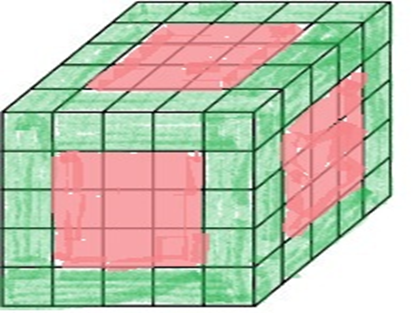1. All the corner cubes are painted green. So there are 8 cubes with 3 sides painted with green.
2. There is no cube having one face red and an adjacent face green as all the green painted cubes got paint on at least 2 faces.
3. Let us calculate the number of cubes with no painting. By formula, = = 27

Therefore, there are 125 – 27 = 98 cubes having at least one face coloured.

1. From the total cubes, Let us subtract the cubes with red painting, cubes with no painting.125 – (9 x 6) – 27 = 44

To explore Clocks in detail, check at the linked article.

Question 6:

Directions: One hundred and twenty-five cubes of the same size are arranged in the form of a cube on a table. Then a column of five cubes is removed from each of the four corners. All the exposed faces of the rest of the solid (except the face touching the table) are coloured red. Now, answer these questions based on the above statement:

1. How many small cubes are there in the solid after the removal of the columns?
2. How many cubes do not have any coloured face?
3. How many cubes have only one red face each?
4. How many cubes have two coloured faces each?
5. How many cubes have more than 3 coloured faces each?

Solution: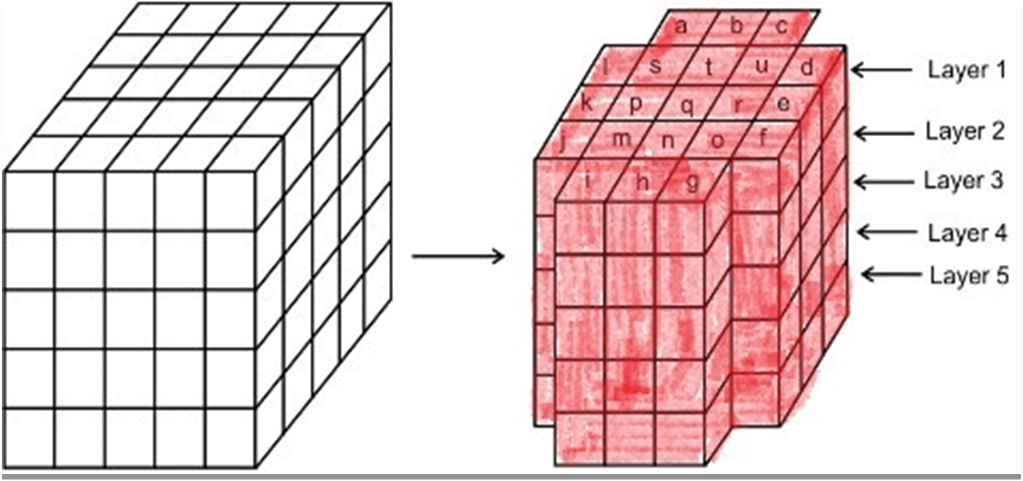1. Since out of 125 total number of cubes, we removed 4 columns of 5 cubes each, the remaining number of cubes = 125 – (4 x 5) = 125 – 20 = 105.
2. Cubes with no paintings lie in the middle. So cubes which are below the cubes named as s, t, u, p, q, r, m, n, o got no painting. Since there are 4 rows below the top layer, total cubes with no painting are (9 x 4) = 36.
3. There are 9 cubes named as m, n, o, p, q, r, s, t and u in layer 1, and 4 cubes (in columns b, e, h, and k) in each of the layers 2, 3, 4 and 5 got one red face. Thus, there are 9 + (4 x 4) = 25 cubes.
4. The columns (a, c, d, f, g, i, j, l) each got 4 cubes in the layers 2, 3, 4, 5. Also in layer 1, h, k, b, e cubes got 2 faces coloured. so total cubes are 32 + 4 = 36
5. There is no cube in the block having more than three coloured faces. There are 8 cubes (in the columns a, c, d, f, g, i, j and l) in layer 1 which have 3 coloured faces. Thus, there are 8 such cubes.